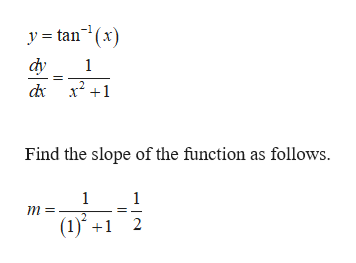# Find an equation for the line tangent to the graph of y=tan-1(x) at the point (1,π/4).

Question

Find an equation for the line tangent to the graph of y=tan-1(x) at the point (1,π/4).

check_circleExpert Solution
Step 1

Given information:

Step 2

Find the equation for tange...help_outlineImage Transcriptionclosey tan(x) dy 1 Find the slope of the function as follows. 1 1 (1) 2 fullscreen

### Want to see the full answer?

See Solution

#### Want to see this answer and more?

Solutions are written by subject experts who are available 24/7. Questions are typically answered within 1 hour*

See Solution
*Response times may vary by subject and question
Tagged in

### Other